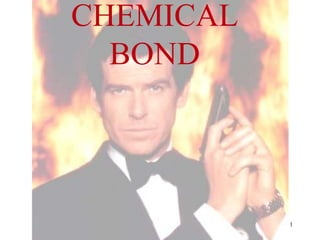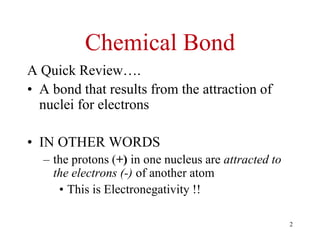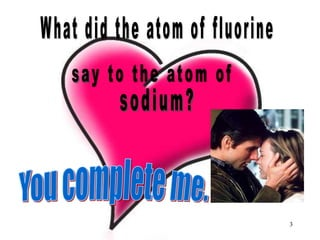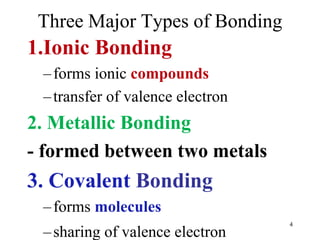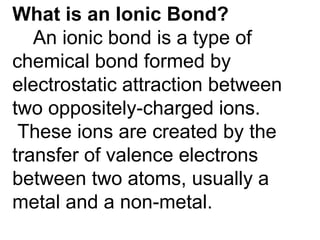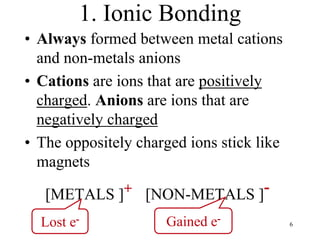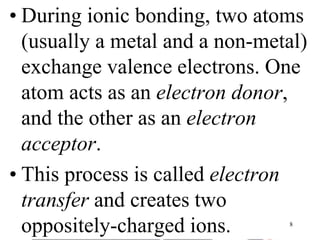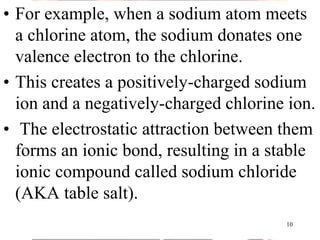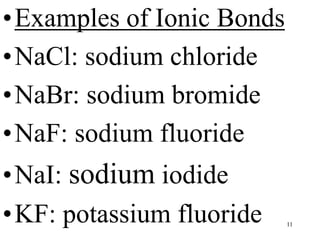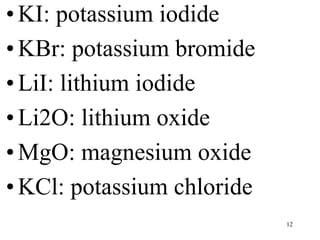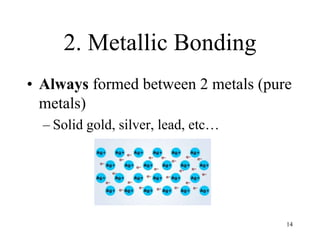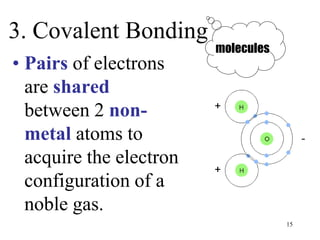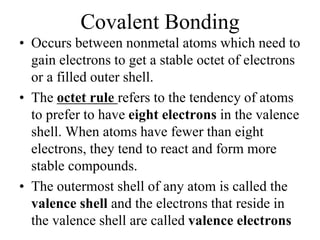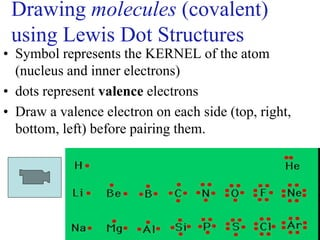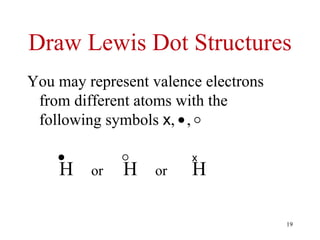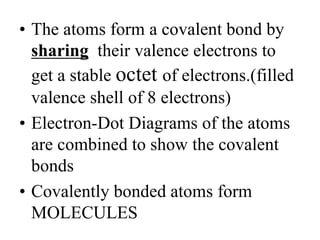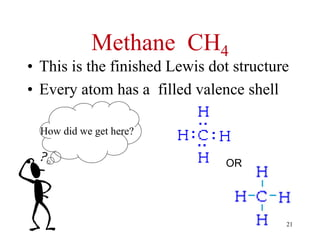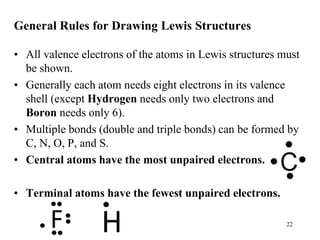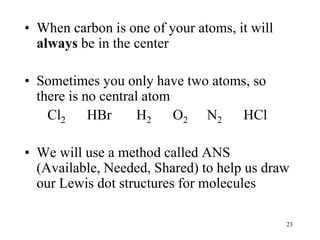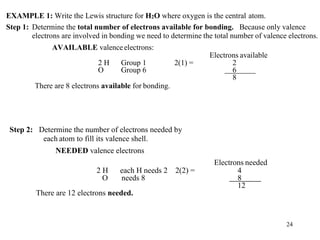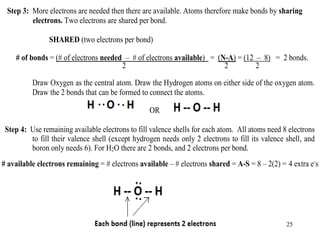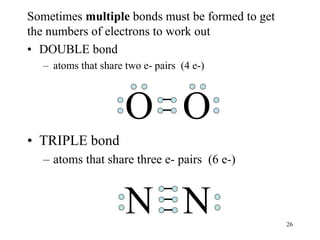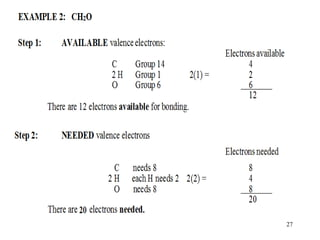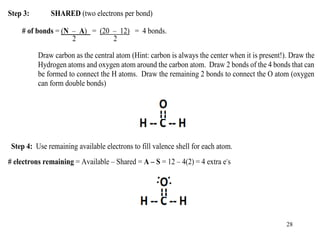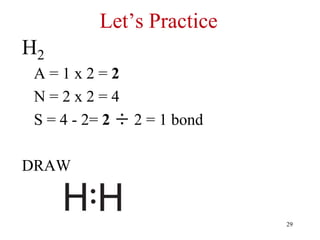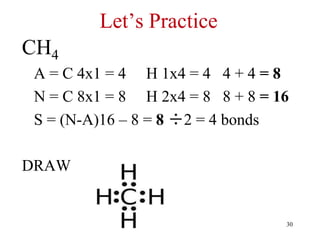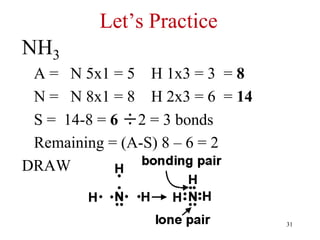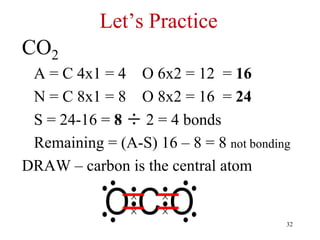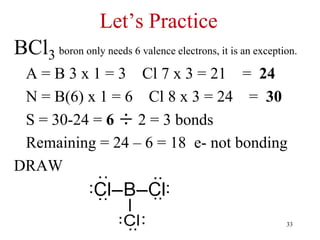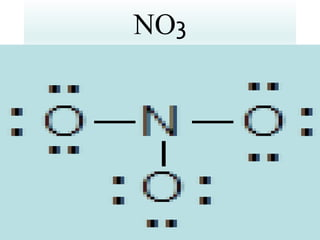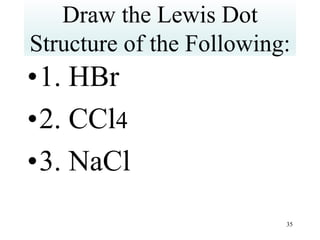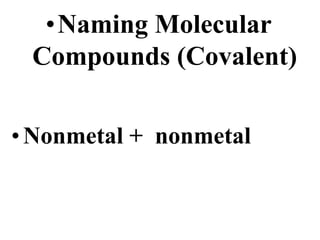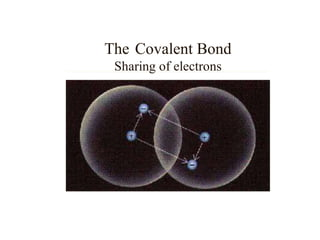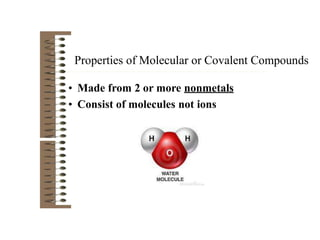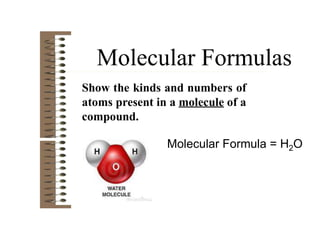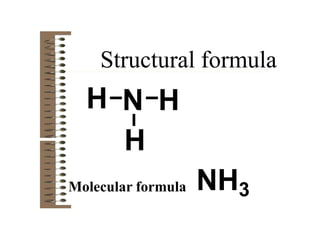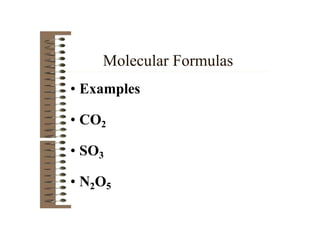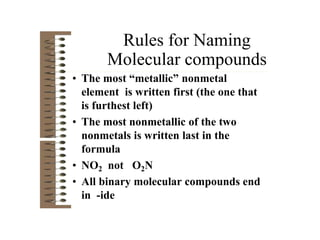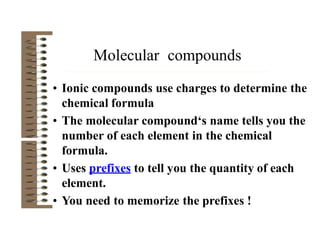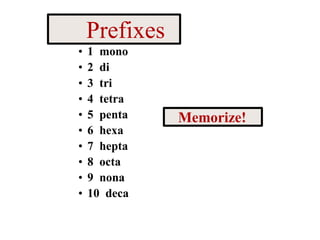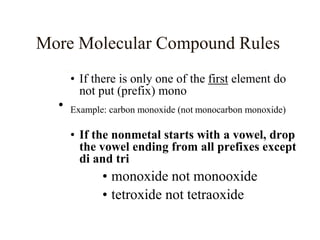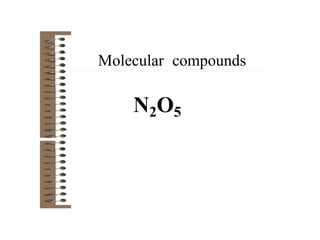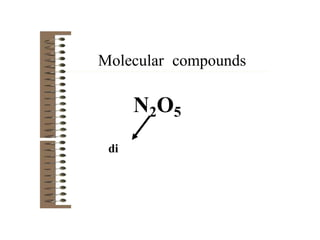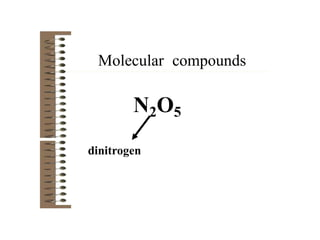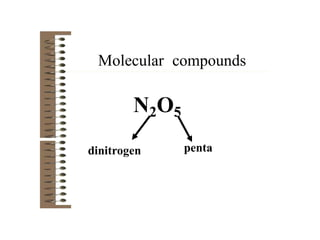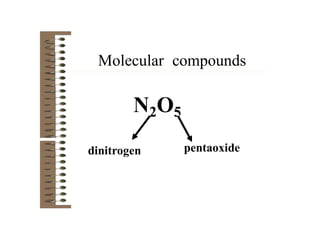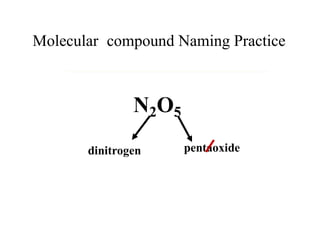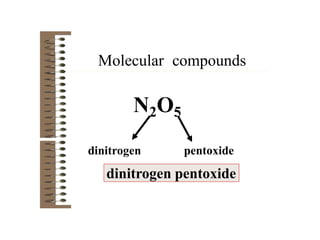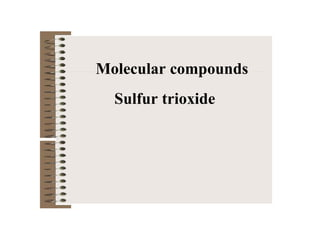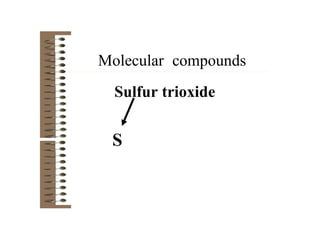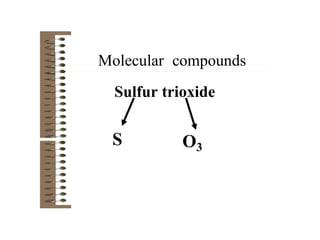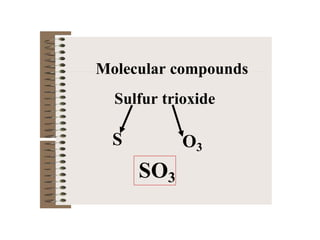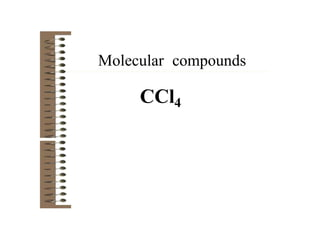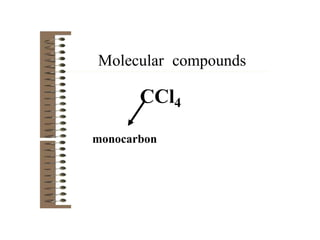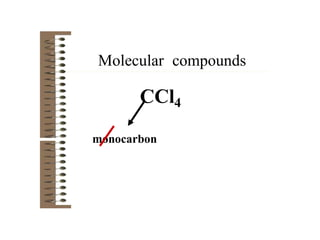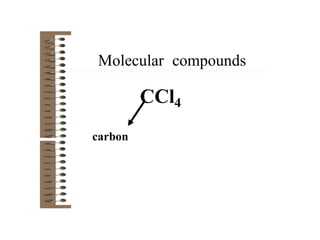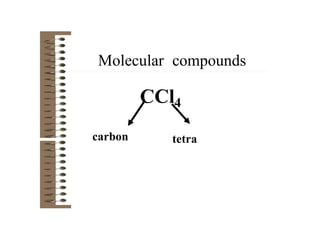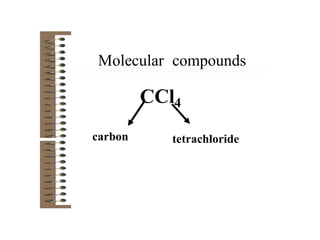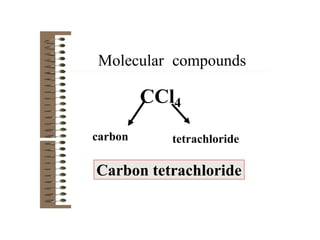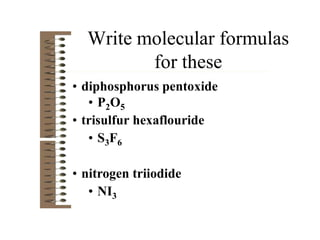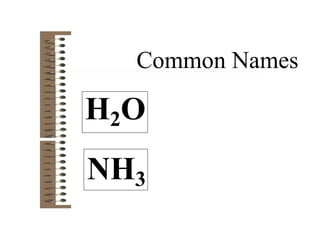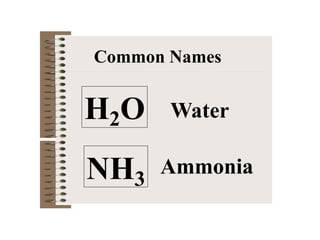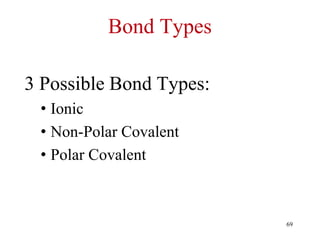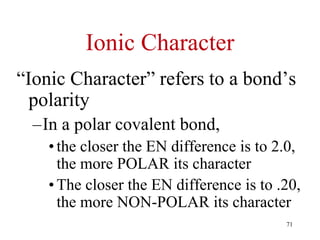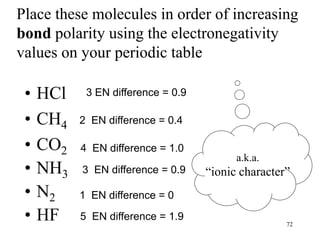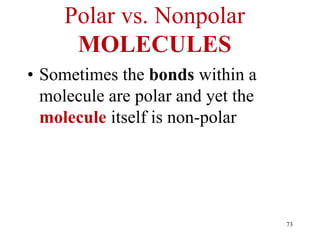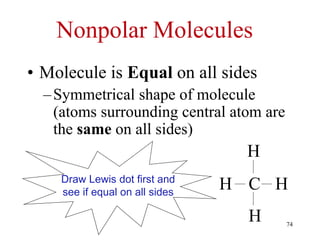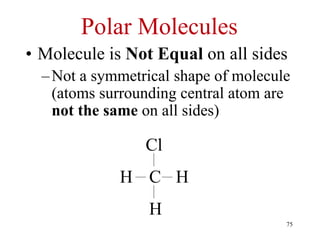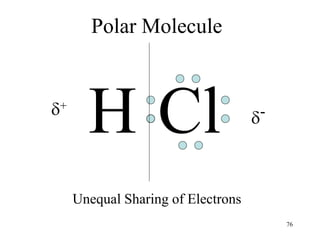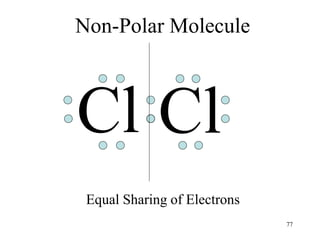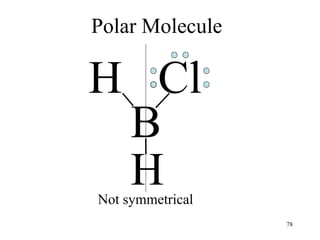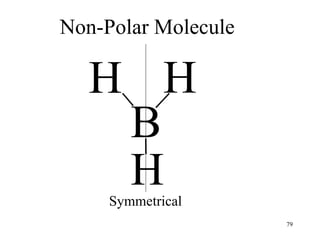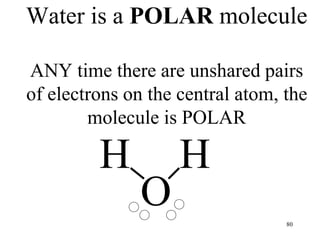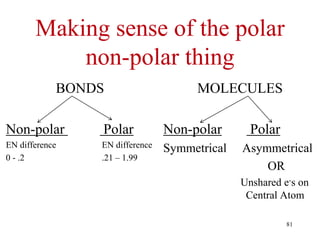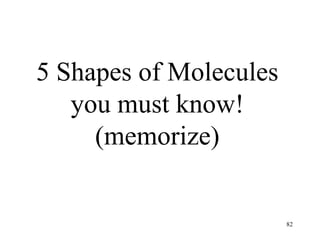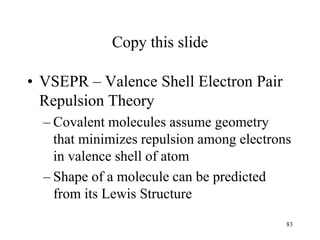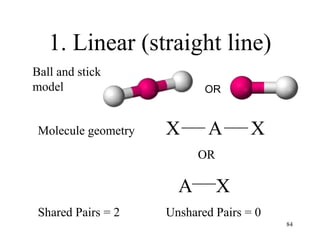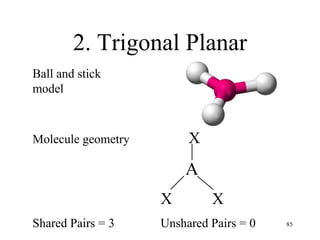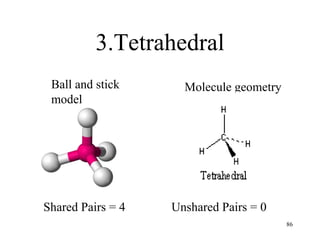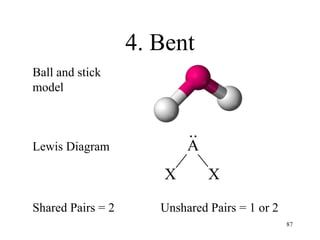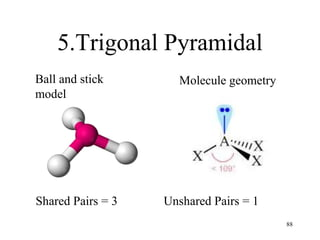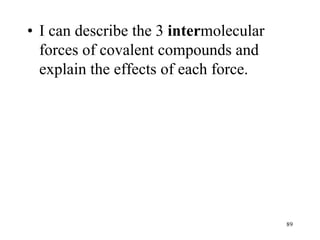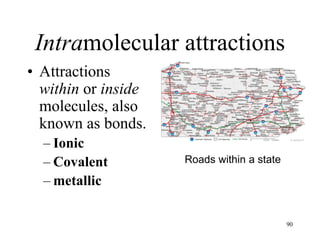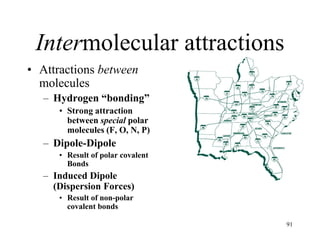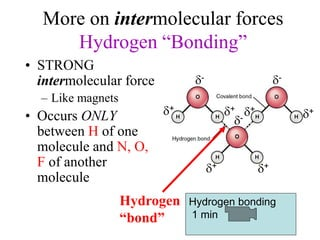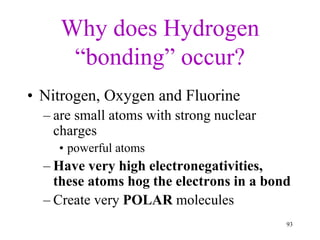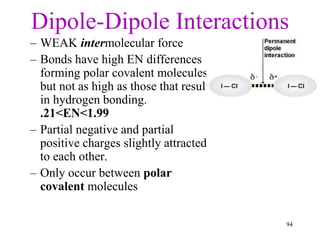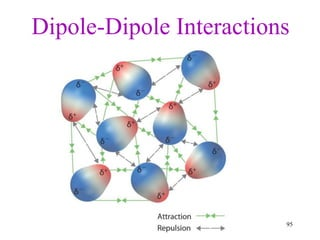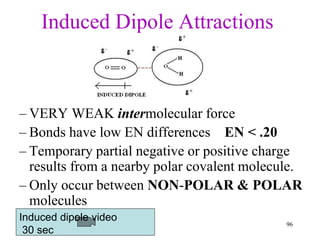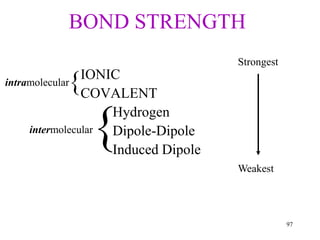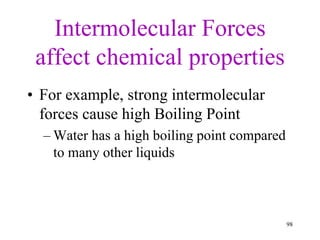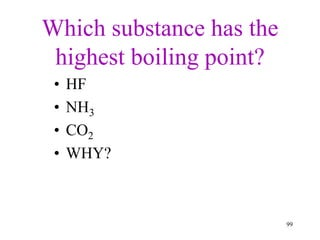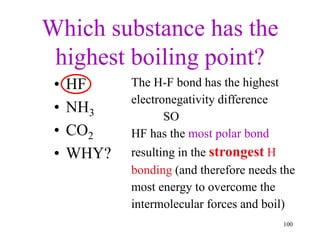1 de 97

### Covalent Bonding.pptx

1. CHEMICAL BOND 1
2. Chemical Bond A Quick Review…. • A bond that results from the attraction of nuclei for electrons • IN OTHER WORDS – the protons (+) in one nucleus are attracted to the electrons (-) of another atom • This is Electronegativity !! 2
3. 3
4. Three Major Types of Bonding 1.Ionic Bonding –forms ionic compounds –transfer of valence electron 2. Metallic Bonding - formed between two metals 3. Covalent Bonding –forms molecules –sharing of valence electron 4
5. 5 What is an Ionic Bond? An ionic bond is a type of chemical bond formed by electrostatic attraction between two oppositely-charged ions. These ions are created by the transfer of valence electrons between two atoms, usually a metal and a non-metal.
6. 1. Ionic Bonding • Always formed between metal cations and non-metals anions • Cations are ions that are positively charged. Anions are ions that are negatively charged • The oppositely charged ions stick like magnets [METALS ]+ [NON-METALS ]- Lost e- Gained e- 6
7. • During ionic bonding, two atoms (usually a metal and a non-metal) exchange valence electrons. One atom acts as an electron donor, and the other as an electron acceptor. • This process is called electron transfer and creates two oppositely-charged ions. 8
8. • For example, when a sodium atom meets a chlorine atom, the sodium donates one valence electron to the chlorine. • This creates a positively-charged sodium ion and a negatively-charged chlorine ion. • The electrostatic attraction between them forms an ionic bond, resulting in a stable ionic compound called sodium chloride (AKA table salt). 10
9. •Examples of Ionic Bonds •NaCl: sodium chloride •NaBr: sodium bromide •NaF: sodium fluoride •NaI: sodium iodide •KF: potassium fluoride 11
10. •KI: potassium iodide •KBr: potassium bromide •LiI: lithium iodide •Li2O: lithium oxide •MgO: magnesium oxide •KCl: potassium chloride 12
11. 2. Metallic Bonding • Always formed between 2 metals (pure metals) – Solid gold, silver, lead, etc… 14
12. 3. Covalent Bonding • Pairs of electrons are shared between 2 non- metal atoms to acquire the electron configuration of a noble gas. molecules 15
13. Covalent Bonding • Occurs between nonmetal atoms which need to gain electrons to get a stable octet of electrons or a filled outer shell. • The octet rule refers to the tendency of atoms to prefer to have eight electrons in the valence shell. When atoms have fewer than eight electrons, they tend to react and form more stable compounds. • The outermost shell of any atom is called the valence shell and the electrons that reside in the valence shell are called valence electrons
14. Drawing molecules (covalent) using Lewis Dot Structures • Symbol represents the KERNEL of the atom (nucleus and inner electrons) • dots represent valence electrons • Draw a valence electron on each side (top, right, bottom, left) before pairing them. 17
15. Always remember atoms are trying to complete their valence shell! “2 will do but 8 is great!” The number of electrons the atom needs is the total number of bonds they can make. Ex. … H? O? F? N? Cl? C? one two one three one four 18
16. Draw Lewis Dot Structures You may represent valence electrons from different atoms with the following symbols x, , H or H or H x 19
17. • The atoms form a covalent bond by sharing their valence electrons to get a stable octet of electrons.(filled valence shell of 8 electrons) • Electron-Dot Diagrams of the atoms are combined to show the covalent bonds • Covalently bonded atoms form MOLECULES
18. Methane CH4 • This is the finished Lewis dot structure • Every atom has a filled valence shell How did we get here? OR 21
19. General Rules for Drawing Lewis Structures • All valence electrons of the atoms in Lewis structures must be shown. • Generally each atom needs eight electrons in its valence shell (except Hydrogen needs only two electrons and Boron needs only 6). • Multiple bonds (double and triple bonds) can be formed by C, N, O, P, and S. • Central atoms have the most unpaired electrons. • Terminal atoms have the fewest unpaired electrons. 22
20. • When carbon is one of your atoms, it will always be in the center • Sometimes you only have two atoms, so there is no central atom Cl2 HBr H2 O2 N2 HCl • We will use a method called ANS (Available, Needed, Shared) to help us draw our Lewis dot structures for molecules 23
21. EXAMPLE 1: Write the Lewis structure for H2O where oxygen is the central atom. Step 1: Determine the total number of electrons available for bonding. Because only valence electrons are involved in bonding we need to determine the total number of valence electrons. AVAILABLE valenceelectrons: Electrons available 2 H Group 1 2(1) = 2 O Group 6 6 8 There are 8 electrons available for bonding. Step 2: Determine the number of electrons needed by each atom to fill its valence shell. NEEDED valence electrons Electrons needed 2 H each H needs 2 2(2) = 4 O needs 8 8 12 There are 12 electrons needed. 24
22. Step 3: More electrons are needed then there are available. Atoms therefore make bonds by sharing electrons. Two electrons are shared per bond. SHARED (two electrons per bond) # of bonds = (# of electrons needed – # of electrons available) = (N-A) = (12 – 8) = 2 bonds. 2 2 2 Draw Oxygen as the central atom. Draw the Hydrogen atoms on either side of the oxygen atom. Draw the 2 bonds that can be formed to connect the atoms. OR Step 4: Use remaining available electrons to fill valence shells for each atom. All atoms need 8 electrons to fill their valence shell (except hydrogen needs only 2 electrons to fill its valence shell, and boron only needs 6). For H2O there are 2 bonds, and 2 electrons per bond. # available electrons remaining = # electrons available – # electrons shared = A-S = 8 – 2(2) = 4 extra e- s 25
23. Sometimes multiple bonds must be formed to get the numbers of electrons to work out • DOUBLE bond – atoms that share two e- pairs (4 e-) O O • TRIPLE bond – atoms that share three e- pairs (6 e-) N N 26
24. 27
25. Step 3: SHARED (two electrons per bond) # of bonds = (N – A) = (20 – 12) = 4 bonds. 2 2 Draw carbon as the central atom (Hint: carbon is always the center when it is present!). Draw the Hydrogen atoms and oxygen atom around the carbon atom. Draw 2 bonds of the 4 bonds that can be formed to connect the H atoms. Draw the remaining 2 bonds to connect the O atom (oxygen can form double bonds) Step 4: Use remaining available electrons to fill valence shell for each atom. # electrons remaining = Available – Shared = A – S = 12 – 4(2) = 4 extra e- s 28
26. Let’s Practice H2 A = 1 x 2 = 2 N = 2 x 2 = 4 S = 4 - 2= 2 ÷ 2 = 1 bond Remaining = A – S = 2 – 2 = 0 DRAW 29
27. Let’s Practice CH4 A = C 4x1 = 4 H 1x4 = 4 4 + 4 = 8 N = C 8x1 = 8 H 2x4 = 8 8 + 8 = 16 S = (N-A)16 – 8 = 8 ÷2 = 4 bonds Remaining = A-S = 8 – 8 = 0 DRAW 30
28. Let’s Practice NH3 A = N 5x1 = 5 H 1x3 = 3 = 8 N = N 8x1 = 8 H 2x3 = 6 = 14 S = 14-8 = 6 ÷2 = 3 bonds Remaining = (A-S) 8 – 6 = 2 DRAW 31
29. Let’s Practice CO2 A = C 4x1 = 4 O 6x2 = 12 = 16 N = C 8x1 = 8 O 8x2 = 16 = 24 S = 24-16 = 8 ÷ 2 = 4 bonds Remaining = (A-S) 16 – 8 = 8 not bonding DRAW – carbon is the central atom 32
30. Let’s Practice BCl3 boron only needs 6 valence electrons, it is an exception. A = B 3 x 1 = 3 Cl 7 x 3 = 21 = 24 N = B(6) x 1 = 6 Cl 8 x 3 = 24 = 30 S = 30-24 = 6 ÷ 2 = 3 bonds Remaining = 24 – 6 = 18 e- not bonding DRAW 33
31. NOꝫ 34
32. •1. HBr •2. CCl4 •3. NaCl 35 Draw the Lewis Dot Structure of the Following:
33. •Naming Molecular Compounds (Covalent) •Nonmetal + nonmetal
34. The Covalent Bond Sharing of electrons
35. Properties of Molecular or Covalent Compounds • Made from 2 or more nonmetals • Consist of molecules not ions
36. Molecular Formulas Show the kinds and numbers of atoms present in a molecule of a compound. Molecular Formula = H2O
37. H N H H NH3 Structural formula Molecular formula
38. Molecular Formulas • Examples • CO2 • SO3 • N2O5
39. Rules for Naming Molecular compounds • The most “metallic” nonmetal element is written first (the one that is furthest left) • The most nonmetallic of the two nonmetals is written last in the formula • NO2 not O2N • All binary molecular compounds end in -ide
40. • Ionic compounds use charges to determine the chemical formula • The molecular compound‘s name tells you the number of each element in the chemical formula. • Uses prefixes to tell you the quantity of each element. • You need to memorize the prefixes ! Molecular compounds
41. Prefixes • 1 mono • 2 di • 3 tri • 4 tetra • 5 penta • 6 hexa • 7 hepta • 8 octa • 9 nona • 10 deca Memorize!
42. • If there is only one of the first element do not put (prefix) mono • Example: carbon monoxide (not monocarbon monoxide) • If the nonmetal starts with a vowel, drop the vowel ending from all prefixes except di and tri • monoxide not monooxide • tetroxide not tetraoxide More Molecular Compound Rules
43. N2O5 Molecular compounds
44. N2O5 Molecular compounds di
45. N2O5 dinitrogen Molecular compounds
46. N2O5 dinitrogen Molecular compounds penta
47. N2O5 dinitrogen Molecular compounds pentaoxide
48. N2O5 dinitrogen Molecular compound Naming Practice pentaoxide
49. N2O5 dinitrogen pentoxide Molecular compounds dinitrogen pentoxide
50. Molecular compounds Sulfur trioxide
51. Molecular compounds Sulfur trioxide S
52. Molecular compounds Sulfur trioxide S
53. Molecular compounds Sulfur trioxide S O3
54. Molecular compounds Sulfur trioxide S O3 SO3
55. Molecular compounds CCl4
56. Molecular compounds CCl4 monocarbon
57. Molecular compounds CCl4 monocarbon
58. Molecular compounds CCl4 carbon
59. Molecular compounds CCl4 tetra carbon
60. Molecular compounds CCl4 tetrachloride carbon
61. Molecular compounds Carbon tetrachloride CCl4 tetrachloride carbon
62. Write molecular formulas for these • diphosphorus pentoxide • P2O5 • trisulfur hexaflouride • S3F6 • nitrogen triiodide • NI3
63. H2O NH3 Common Names
64. H2O NH3 Water Ammonia Common Names
65. 68
66. Bond Types 3 Possible Bond Types: • Ionic • Non-Polar Covalent • Polar Covalent 69
67. Ionic Character “Ionic Character” refers to a bond’s polarity –In a polar covalent bond, •the closer the EN difference is to 2.0, the more POLAR its character •The closer the EN difference is to .20, the more NON-POLAR its character 71
68. Place these molecules in order of increasing bond polarity using the electronegativity values on your periodic table • HCl • CH4 • CO2 • NH3 • N2 • HF a.k.a. “ionic character” 72 1 EN difference = 0 2 EN difference = 0.4 3 EN difference = 0.9 4 EN difference = 1.0 3 EN difference = 0.9 5 EN difference = 1.9
69. Polar vs. Nonpolar MOLECULES • Sometimes the bonds within a molecule are polar and yet the molecule itself is non-polar 73
70. Nonpolar Molecules • Molecule is Equal on all sides –Symmetrical shape of molecule (atoms surrounding central atom are the same on all sides) H H H H C Draw Lewis dot first and see if equal on all sides 74
71. Polar Molecules • Molecule is Not Equal on all sides –Not a symmetrical shape of molecule (atoms surrounding central atom are not the same on all sides) Cl H H H C 75
72. H Cl - + Polar Molecule Unequal Sharing of Electrons 76
73. Cl Non-Polar Molecule Cl Equal Sharing of Electrons 77
74. H Cl Polar Molecule Not symmetrical H B 78
75. H H Non-Polar Molecule Symmetrical H B 79
76. H H O Water is a POLAR molecule ANY time there are unshared pairs of electrons on the central atom, the molecule is POLAR 80
77. Making sense of the polar non-polar thing BONDS Non-polar Polar EN difference EN difference 0 - .2 .21 – 1.99 MOLECULES Non-polar Polar Symmetrical Asymmetrical OR Unshared e-s on Central Atom 81
78. 5 Shapes of Molecules you must know! (memorize) 82
79. Copy this slide • VSEPR – Valence Shell Electron Pair Repulsion Theory – Covalent molecules assume geometry that minimizes repulsion among electrons in valence shell of atom – Shape of a molecule can be predicted from its Lewis Structure 83
80. 1. Linear (straight line) Ball and stick model Molecule geometry X A X OR A X Shared Pairs = 2 Unshared Pairs = 0 OR 84
81. 2. Trigonal Planar Ball and stick model Molecule geometry X A X X Shared Pairs = 3 Unshared Pairs = 0 85
82. 3.Tetrahedral Ball and stick model Molecule geometry Shared Pairs = 4 Unshared Pairs = 0 86
83. 4. Bent Ball and stick model Lewis Diagram A X X .. Shared Pairs = 2 Unshared Pairs = 1 or 2 87
84. 5.Trigonal Pyramidal Ball and stick model Molecule geometry Shared Pairs = 3 Unshared Pairs = 1 88
85. • I can describe the 3 intermolecular forces of covalent compounds and explain the effects of each force. 89
86. • Attractions within or inside molecules, also known as bonds. – Ionic – Covalent – metallic Intramolecular attractions 90 Roads within a state
87. • Attractions between molecules – Hydrogen “bonding” • Strong attraction between special polar molecules (F, O, N, P) – Dipole-Dipole • Result of polar covalent Bonds – Induced Dipole (Dispersion Forces) • Result of non-polar covalent bonds Intermolecular attractions 91
88. More on intermolecular forces Hydrogen “Bonding” • STRONG intermolecular force – Like magnets • Occurs ONLY between H of one molecule and N, O, F of another molecule Hydrogen “bond” - + + - + + + + - 92 Hydrogen bonding 1 min
89. Why does Hydrogen “bonding” occur? • Nitrogen, Oxygen and Fluorine – are small atoms with strong nuclear charges • powerful atoms – Have very high electronegativities, these atoms hog the electrons in a bond – Create very POLAR molecules 93
90. Dipole-Dipole Interactions – WEAK intermolecular force – Bonds have high EN differences forming polar covalent molecules, but not as high as those that result in hydrogen bonding. .21<EN<1.99 – Partial negative and partial positive charges slightly attracted to each other. – Only occur between polar covalent molecules 94
91. Dipole-Dipole Interactions 95
92. Induced Dipole Attractions – VERY WEAK intermolecular force – Bonds have low EN differences EN < .20 – Temporary partial negative or positive charge results from a nearby polar covalent molecule. – Only occur between NON-POLAR & POLAR molecules 96 Induced dipole video 30 sec
93. BOND STRENGTH IONIC COVALENT Hydrogen Dipole-Dipole Induced Dipole   intramolecular intermolecular Strongest Weakest 97
94. Intermolecular Forces affect chemical properties • For example, strong intermolecular forces cause high Boiling Point – Water has a high boiling point compared to many other liquids 98
95. Which substance has the highest boiling point? • HF • NH3 • CO2 • WHY? 99
96. Which substance has the highest boiling point? • HF • NH3 • CO2 • WHY? The H-F bond has the highest electronegativity difference SO HF has the most polar bond resulting in the strongest H bonding (and therefore needs the most energy to overcome the intermolecular forces and boil) 100
97. The End 101